Next: Worked example 4.3: Raising Up: Newton's laws of motion Previous: Worked example 4.1: In

## Worked example 4.2: Block accelerating up a slope

Question: Consider the diagram. Suppose that the block, mass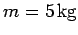, is subject to a horizontal force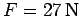. What is the acceleration of the block up the (frictionless) slope?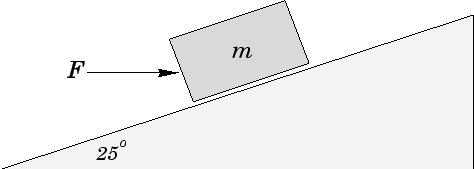Answer: Only that component of the applied force which is parallel to the incline has any influence on the block's motion: the normal component of the applied force is canceled out by the normal reaction of the incline. The component of the applied force acting up the incline is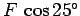. Likewise, the component of the block's weight acting down the incline is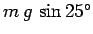. Hence, using Newton's second law to determine the accelerationof the block up the incline, we obtain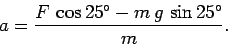Sinceand, we have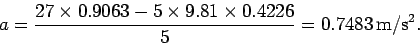Richard Fitzpatrick 2006-02-02